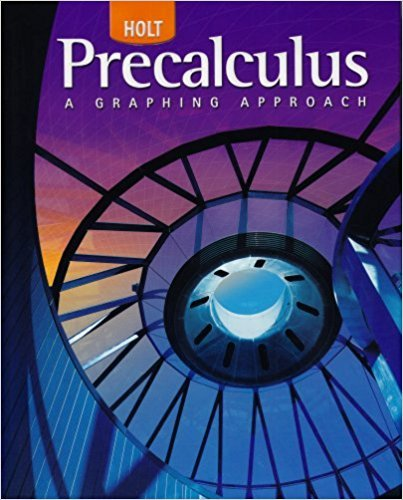×
×

# Solutions for Chapter 2.5: Inequalities## Full solutions for Precalculus | 1st Edition

ISBN: 9780030416477Solutions for Chapter 2.5: Inequalities

Solutions for Chapter 2.5
4 5 0 367 Reviews
28
2
##### ISBN: 9780030416477

Precalculus was written by and is associated to the ISBN: 9780030416477. This expansive textbook survival guide covers the following chapters and their solutions. This textbook survival guide was created for the textbook: Precalculus, edition: 1. Since 88 problems in chapter 2.5: Inequalities have been answered, more than 23531 students have viewed full step-by-step solutions from this chapter. Chapter 2.5: Inequalities includes 88 full step-by-step solutions.

Key Calculus Terms and definitions covered in this textbook

P(A or B) = P(A) + P(B) - P(A and B). If A and B are mutually exclusive events, then P(A or B) = P(A) + P(B)

• Combination

An arrangement of elements of a set, in which order is not important

• Constant term

See Polynomial function

• Division

a b = aa 1 b b, b Z 0

• Endpoint of an interval

A real number that represents one “end” of an interval.

• Equal complex numbers

Complex numbers whose real parts are equal and whose imaginary parts are equal.

• Frequency distribution

See Frequency table.

• Leaf

The final digit of a number in a stemplot.

• Line graph

A graph of data in which consecutive data points are connected by line segments

• Major axis

The line segment through the foci of an ellipse with endpoints on the ellipse

A measure that tells how widely distributed data are.

• Number line graph of a linear inequality

The graph of the solutions of a linear inequality (in x) on a number line

• Outcomes

The various possible results of an experiment.

• Paraboloid of revolution

A surface generated by rotating a parabola about its line of symmetry.

• Power rule of logarithms

logb Rc = c logb R, R 7 0.

• Pseudo-random numbers

Computer-generated numbers that can be used to approximate true randomness in scientific studies. Since they depend on iterative computer algorithms, they are not truly random

• Quartile

The first quartile is the median of the lower half of a set of data, the second quartile is the median, and the third quartile is the median of the upper half of the data.

• Range (in statistics)

The difference between the greatest and least values in a data set.

• Speed

The magnitude of the velocity vector, given by distance/time.

• Vector

An ordered pair <a, b> of real numbers in the plane, or an ordered triple <a, b, c> of real numbers in space. A vector has both magnitude and direction.

×